Like   Tweet   in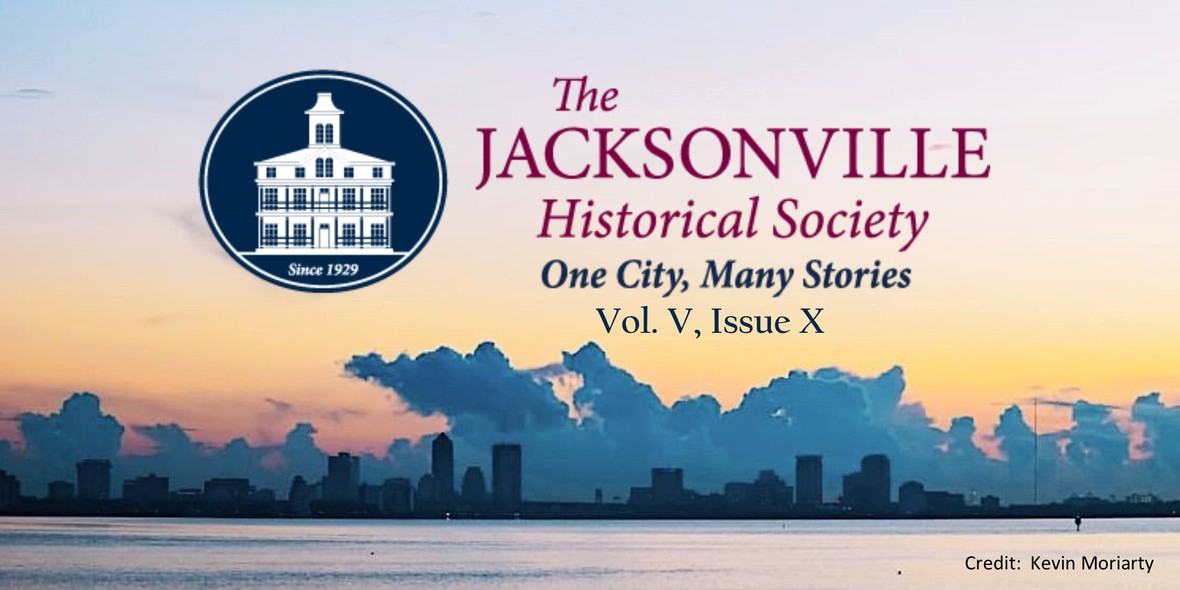# Jacksonville History Matters: NEWS & EVENTS

 table div table+table+table+table div table{width:100%;padding:0}table div table+table+table+table div table img{width:96.23%;padding:0;float:none}table div table+table+table+table div table td{width:100%;padding:0 1.88% 18px}/* styles */## Speaking of Jacksonville History

 /* styles */ Demolition of old buildings has been a lively topic in Jacksonville of late, and the Jacksonville Historical Society is naturally a party to the conversation. The focus is currently on “Fire Station #5,” located at 347 Riverside Ave. Nearly 110 years ago, Fire Station #5 was constructed by the City of Jacksonville, a response to Jacksonville’s Great Fire of 1901, the third largest urban fire in U.S. history. The station was decommissioned by Jacksonville Fire and Rescue in 2008, and has been vacant ever since. Fire Station #5 stands at the intersection of Riverside Avenue and Forest Street. The City of Jacksonville plans to extend Forest Street across the site as part of the redevelopment of the campus of Fidelity National Information Services, and to help accommodate growth in the Brooklyn Neighborhood.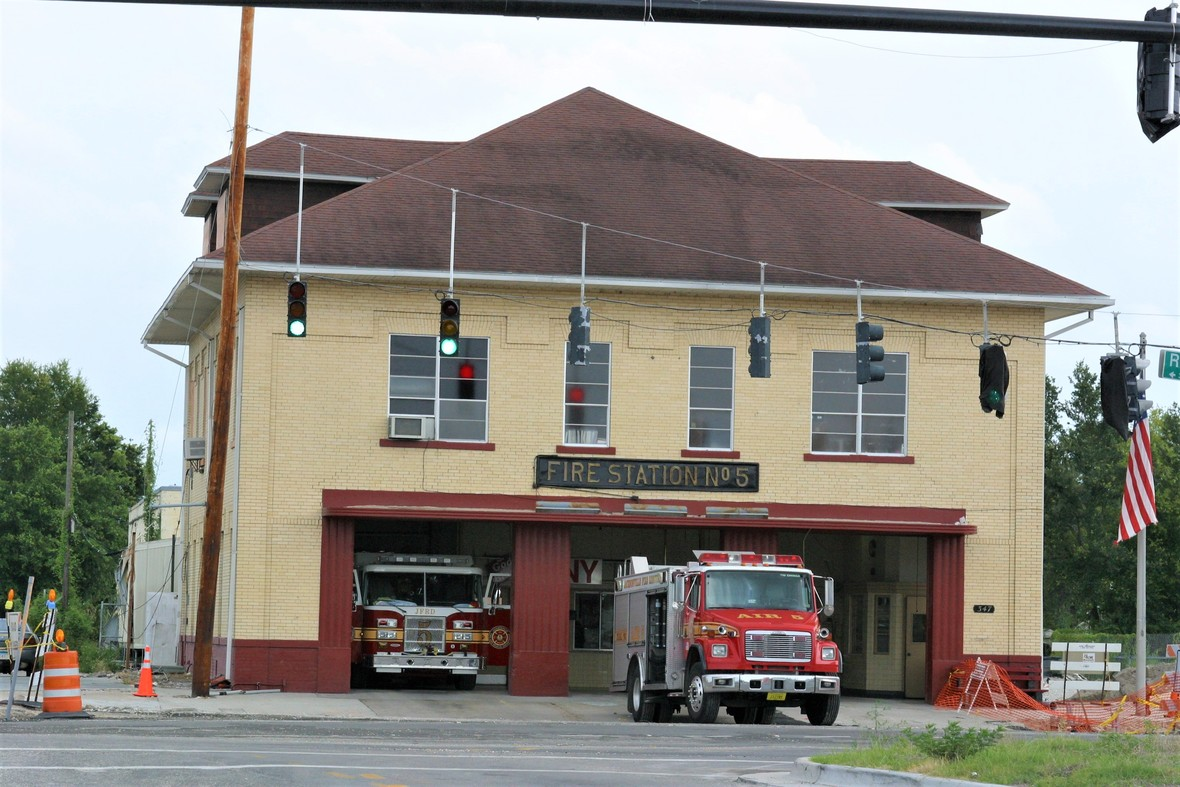JFRD, Fire Station No. 5, 2005. Photo Credit: Ed Booth
 /* styles */ Recently, the Downtown Investment Authority announced that, absent a viable plan to move the building, the old fire station must be demolished. The Jacksonville Historical Society encourages every community stakeholder who shares concern for this historic building to act to preserve it in a new location. As an authentic part of our city’s history, Fire Station #5 lends a sense of place and identity to Jacksonville. Stories about a place help people to recognize who has been there before, what they did, and what happened. It becomes a way to take ownership of a place, as those listeners share the story with others. For many years, the decommissioned Fire Station #5 has been on the Jacksonville Historical Society’s “Most Endangered Buildings List.” The building contributes valuably to telling the story of some of Jacksonville’s most influential decades and events. Countless firefighters, working from this facility, have saved lives and defended our property from other catastrophic events. Their stories are among the many this city holds that deserve preservation and sharing. This big, complicated city’s many fascinating stories are why there is a Jacksonville Historical Society. We encourage flexibility and support on the part of the City and its agencies, for anyone with the passion and resources to relocate this example of Jacksonville’s legacy. As with other structures, this building can be moved and repurposed elsewhere in Brooklyn or Lavilla, where historic authenticity could help activate underused space through creative adaptive reuse. Jacksonville has lost many historic buildings that may otherwise have strengthened Jacksonville’s cultural and historic story. Fire House #5 is worthy of preservation, in order to connect our city’s past with its future. We stand ready to help advance this purpose by connecting people, plans and resources. For an appropriate plan, some funds may be available through the Downtown Investment Authority. Contact the JHS at 904-665-0064 to discuss how we can help. On Jacksonville’s east side, three adjacent houses also face demolition, and offer an opportunity for preservationists and entrepreneurs – or both. The three vintage shotgun houses are in the 500 block of East Duval Street (one is on Washington Street near Duval Street). They have been approved for demolition by the Jacksonville Historic Preservation Commission, and must be relocated. The contact for this property owner is Cyndy K. Trimmer, with the law firm of Driver, McAfee, Hawthorne & Diebenow, PLLC, at ctrimmer@drivermcafee.com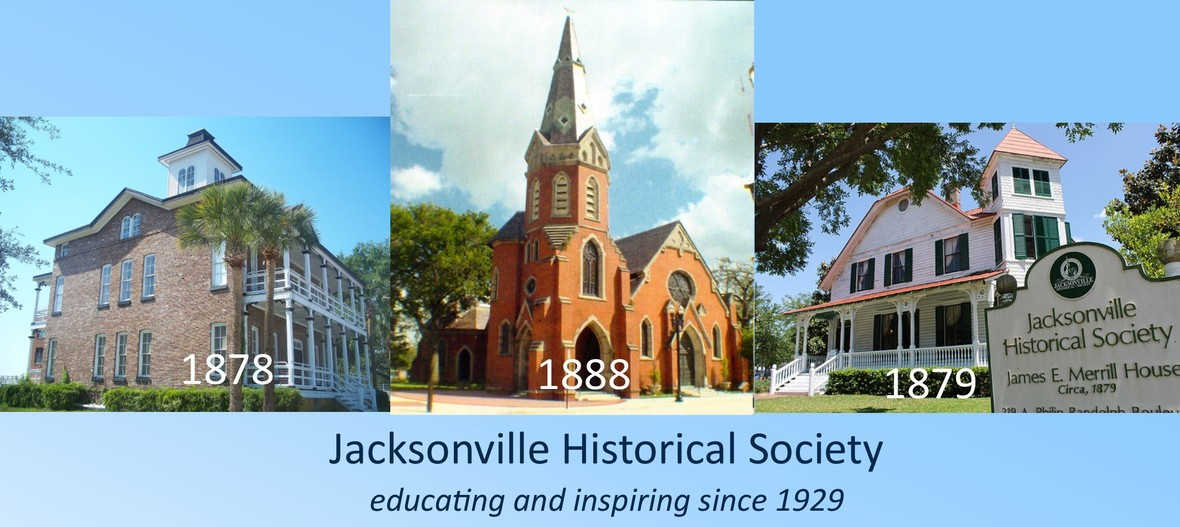/* styles */ Sometimes the Historical Society is asked why it does not directly intervene by purchasing historic buildings that are in need of preservation. The answer is that we already maintain four historic buildings in or near downtown. Two are owned by the City and maintained by the JHS; the other two purchased in 2012 by the JHS. On our budget of slightly over \$250K, we also operate our large and growing archives, employ a professional staff, produce educational programming, and serve all of Jacksonville as the City’s principal citywide history resource. In order to expand our cramped archival storage, processing and research facilities, we are currently raising funds to restore and activate the ca. 1920 Florida Casket Company building, popularly known as the “Casket Factory.” In short, we do all that is possible, making the most efficient use of our resources. We welcome opportunities to support and strengthen historic preservation through collaboration with Jacksonville’s people and institutions. Alan Bliss Executive Director
 table div table+table+table+table+table+table+table+table+table+table+table div table{width:100%;padding:0}table div table+table+table+table+table+table+table+table+table+table+table div table img{width:96.23%;padding:0;float:none}table div table+table+table+table+table+table+table+table+table+table+table div table td{width:100%;padding:0 1.88% 18px}/* styles */## Magic Bell Brings Holiday Cheer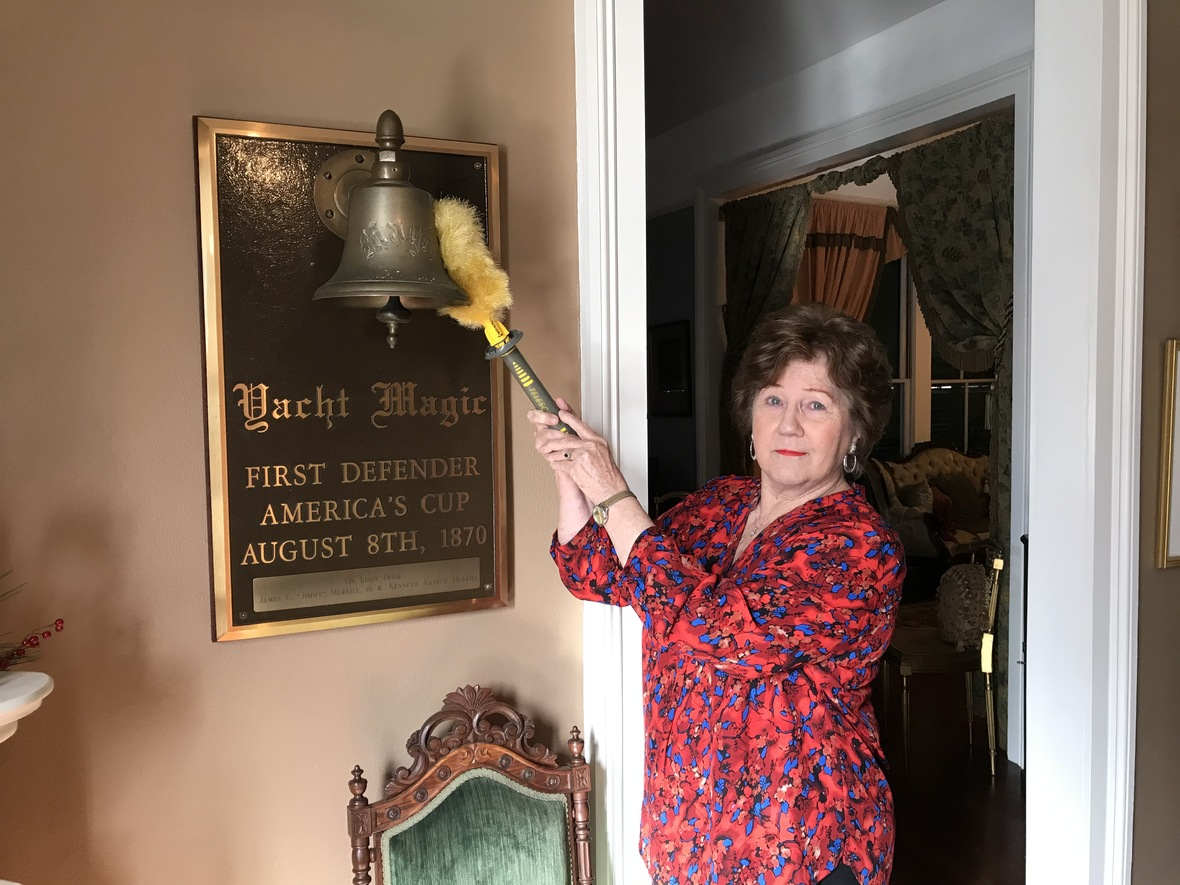Docent Nancy Gandy gives the Merrill House some tender loving care
 /* styles */ Once again, that special time is upon us, when thousands of families and friends pass through Old St. Andrews during our annual Gingerbread Extravaganza. It’s also the perfect opportunity to experience our historic Merrill House next door. If you are one of those individuals who will tour the Merrill House this December, odds are you’ll meet Nancy Gandy, one of our most cherished docents. Few people know the Merrill House as well as Nancy does. Still, her thirst for knowledge seems unquenchable as she continues to uncover the answers to some of the home’s greatest mysteries. Case in point: the bell from Yacht Magic, First Defender of America's Cup. This wonderful artifact hangs in Mr. Merrill’s library next to the fireplace. But how did it get there? Nancy has done a great deal of research to answer that question, and what she’s discovered is fascinating. Designed by Richard Fanning Loper and built in Philadelphia, Magic (then known as Madgie) launched in 1857 as a centerboard sloop for Loper’s personal use. Over the next few years, Madgie underwent a number of modifications to improve her performance. Madgie was eventually sold in 1864, renamed Magic and was soon sailing under the banner of the New York Yacht Club. In 1869, it was sold again to Franklin Osgood, who successfully defended the inaugural America's Cup in 1870 from the English challenger, Cambria.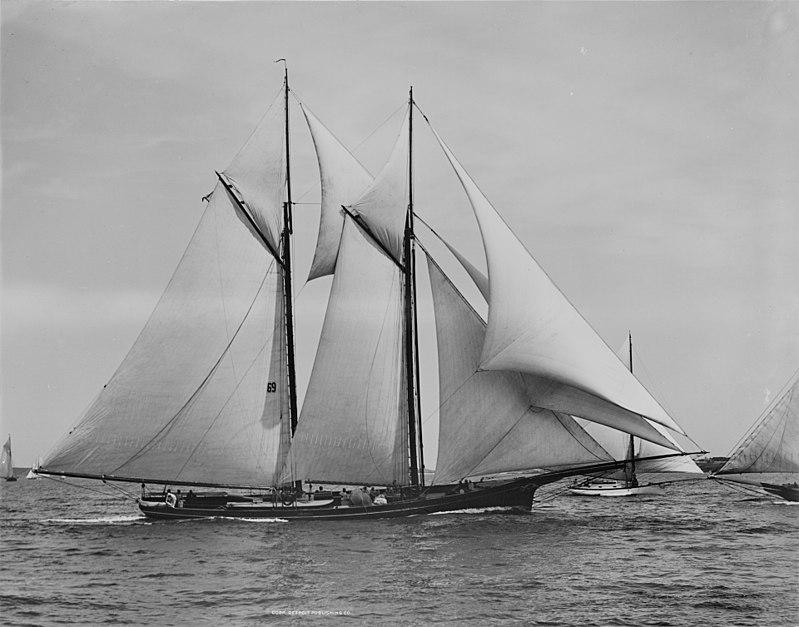"Magic, Cup defender", August 10, 1888 - Detroit Publishing Company photograph collection (Library of Congress)
 /* styles */ Over the years, Magic would sail through the hands of nineteen different owners, including the United States Navy, who used her as a supply craft during the Spanish-American War. It’s after the war that things take an interesting turn for Magic. In 1899, the Navy deposited Magic at the Merrill-Stevens Drydock & Repair, Co. in Jacksonville FL. It would languish there for a year before the shipyard sold it to John Lowe to pay the outstanding bill. The bell was likely kept as a memento at this time. Many years later, the bell was donated by the Merrill family to hang in the historic home as a reminder of Mr. Merrill’s legacy of shipbuilding. It’s interesting to consider that the Magic was sold one year prior to the Great Fire of 1901. Who knows what its fate would have been had it remained in Jacksonville during that terrible event? Nevertheless, Magic began to fall into disrepair and a hurricane in 1926 left it stranded in Key West. At that time, the 69 year old first Defender of America’s Cup was loaded with explosives, dragged offshore and destroyed. A storied end for a storied vessel. If you haven’t experienced a tour of the historic Merrill House, you’re missing something special. You can see the bell in person and everything else the house has to offer during our 17th Annual Gingerbread Extravaganza this December. Tours will be offered throughout the event. We hope to see you there! Mitchell Hemann Head Archivist
 table div table+table+table+table+table+table+table+table+table+table+table+table+table+table+table+table+table div table{width:100%;padding:0}table div table+table+table+table+table+table+table+table+table+table+table+table+table+table+table+table+table div table img{width:96.23%;padding:0;float:none}table div table+table+table+table+table+table+table+table+table+table+table+table+table+table+table+table+table div table td{width:100%;padding:0 1.88% 18px}/* styles */## 17th Annual Gingerbread Extravaganza Opening December 4th!

 /* styles */ You've worked hard all year. It's time to immerse yourself in over 2,000 square feet of gingerbread confection!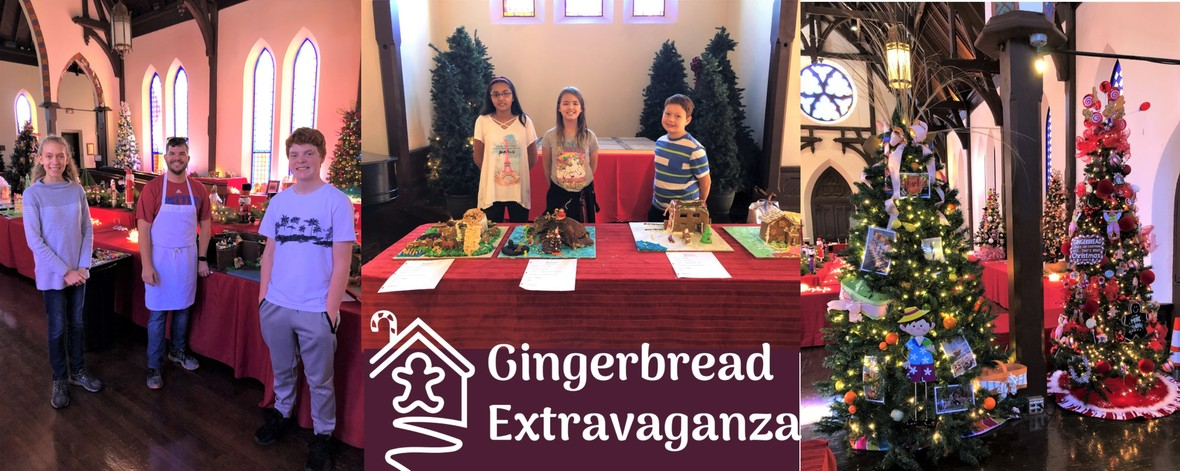Left: Kristen Davis and Kelson Davis assist Chef Phil Abbott (DCPS Peterson Academy Culinary Program). Center: Students from the Keys Educational Resource Center display their entries. Right: Junior League Sustainer historic trees adorn Old St. Andrews.
 /* styles */ The 17th Annual Gingerbread Extravaganza is honored to exhibit dozens of edible structures hand-crafted by local builders, chefs, friends, families, students and organizations! During your Extravaganza experience you can explore edible structures of historic impact, architecture, fantasy and whimsy! Test your skills in the annual Scavenger Hunt, vote for the Peoples’ Choice Awards, explore the Gift Shop, enjoy some hot apple cider and tour the Historic Merrill House at this Jacksonville tradition!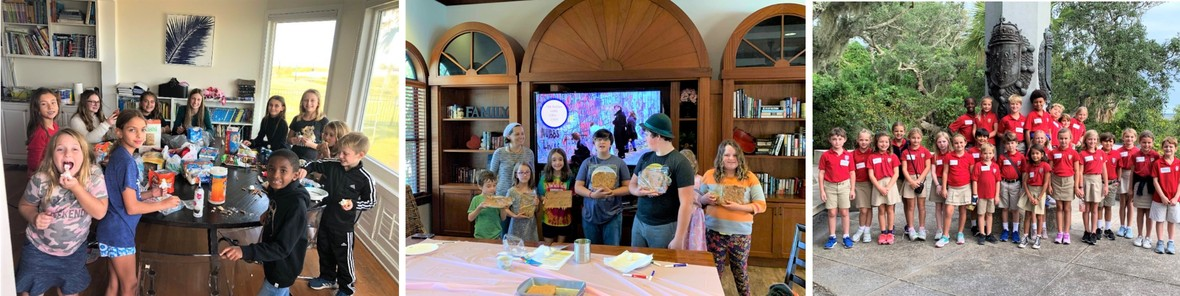Talented Building Teams: (L to R) The Jones Family, Baking History - St. Johns County, St. Mark's Day School Third Grade

# Historic Exhibits at the Gingerbread Extravaganza

The 2019 Gingerbread Extravaganza is honored to celebrate the season with several special exhibits. The Cathedral District will display a six foot replica of the historic Downtown Cathedral District with gingerbread churches marking the inaugural Christmas in the Cathedral District event which showcases the churches and homes in this historic neighborhood. All are invited to join the music, singing, and tours of Christmas in the Cathedral District during the December Art Walk, Wednesday, December 5th, immediately after the first day of the Gingerbread Extravaganza!

In commemoration of the Springfield Historic District's epic sesquicentennial, we welcome the top gingerbread houses from the 33rd Annual Springfield Holiday Tour on loan from Springfield Improvement Association and Archives (SIAA) and Springfield Preservation and Revitalization (SPAR)

Come see these great exhibits and many more at the 2019 Gingerbread Extravaganza!

# Extravaganza Origins

The Gingerbread Extravaganza was launched in 2003 by the South Jacksonville Rotary from the inspiration of member, Joseph Miller (1956 – 2017), a professionally trained culinary arts chef. The South Jacksonville Rotary and the Junior League of Jacksonville are still involved in the Extravaganza today providing valuable time and talent to successfully stage the elaborate month-long event. The proceeds from all this fun and creativity benefit the educational programs and archives of the Jacksonville Historical Society. For dates, details and other fun facts, visit Extravaganza Headquarters here.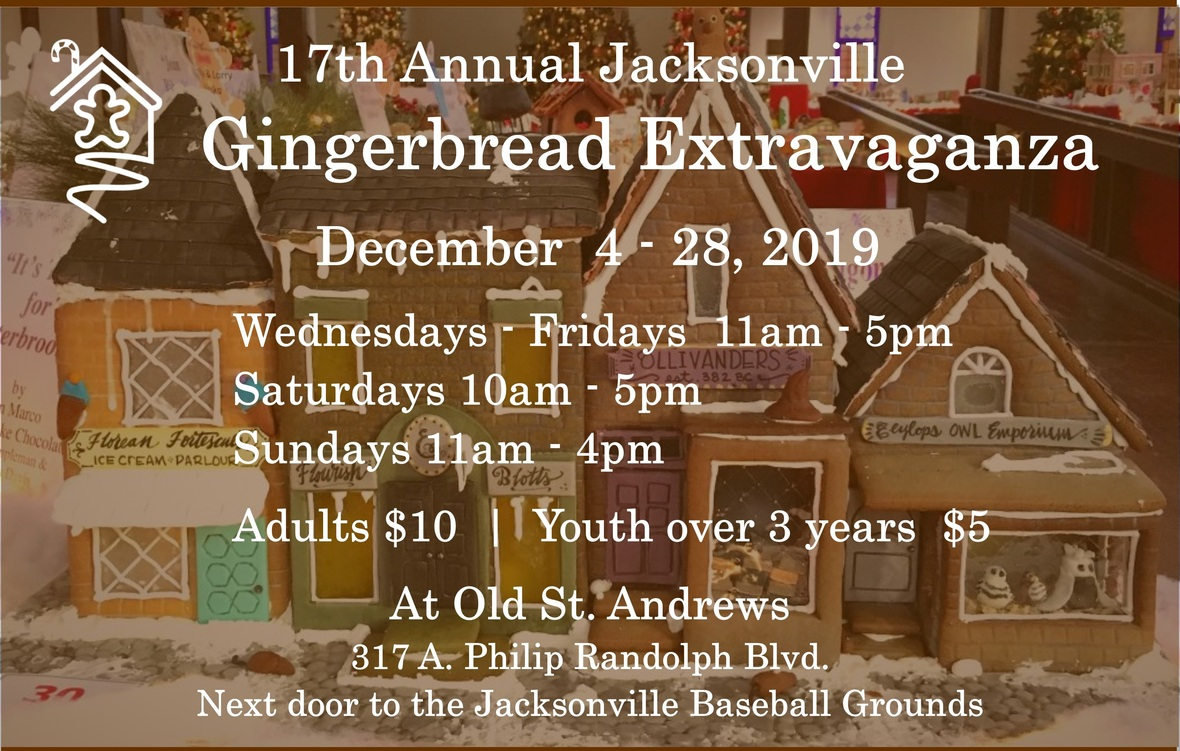table div table+table+table+table+table+table+table+table+table+table+table+table+table+table+table+table+table+table+table+table+table+table+table+table+table+table+table div table{width:100%;padding:0}table div table+table+table+table+table+table+table+table+table+table+table+table+table+table+table+table+table+table+table+table+table+table+table+table+table+table+table div table img{width:96.23%;padding:0;float:none}table div table+table+table+table+table+table+table+table+table+table+table+table+table+table+table+table+table+table+table+table+table+table+table+table+table+table+table div table td{width:100%;padding:0 1.88% 18px}/* styles */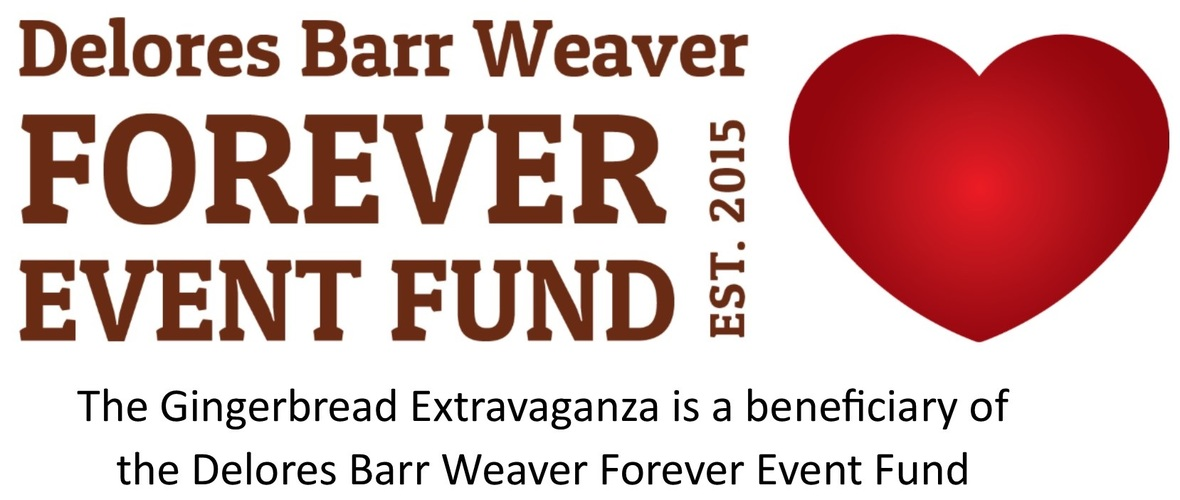table div table+table+table+table+table+table+table+table+table+table+table+table+table+table+table+table+table+table+table+table+table+table+table+table+table+table+table+table+table div table{width:100%;padding:0}table div table+table+table+table+table+table+table+table+table+table+table+table+table+table+table+table+table+table+table+table+table+table+table+table+table+table+table+table+table div table img{width:96.23%;padding:0;float:none}table div table+table+table+table+table+table+table+table+table+table+table+table+table+table+table+table+table+table+table+table+table+table+table+table+table+table+table+table+table div table td{width:100%;padding:0 1.88% 18px}/* styles */# Vystar Members are invited to Vystar Sundays at the Gingerbread Extravaganza!

Free admission for Vystar members on Sunday, December 15 and December 22 when you present your Vystar Member card! Thank you to Vystar Credit Union for their generous support of the 2019 Gingerbread Extravaganza!

 table div table+table+table+table+table+table+table+table+table+table+table+table+table+table+table+table+table+table+table+table+table+table+table+table+table+table+table+table+table+table+table div table{width:100%;padding:0}table div table+table+table+table+table+table+table+table+table+table+table+table+table+table+table+table+table+table+table+table+table+table+table+table+table+table+table+table+table+table+table div table img{width:96.23%;padding:0;float:none}table div table+table+table+table+table+table+table+table+table+table+table+table+table+table+table+table+table+table+table+table+table+table+table+table+table+table+table+table+table+table+table div table td{width:100%;padding:0 1.88% 18px}/* styles */## Accepting Gently Used Holiday Treasures

 table div table+table+table+table+table+table+table+table+table+table+table+table+table+table+table+table+table+table+table+table+table+table+table+table+table+table+table+table+table+table+table+table+table div table,table.module-32{width:32.08%;float:right;padding:0}table div table+table+table+table+table+table+table+table+table+table+table+table+table+table+table+table+table+table+table+table+table+table+table+table+table+table+table+table+table+table+table+table+table div table a{border:0 none;text-decoration:none}table div table+table+table+table+table+table+table+table+table+table+table+table+table+table+table+table+table+table+table+table+table+table+table+table+table+table+table+table+table+table+table+table+table div table img{width:100%!important;border:0 none;text-decoration:none}table div table+table+table+table+table+table+table+table+table+table+table+table+table+table+table+table+table+table+table+table+table+table+table+table+table+table+table+table+table+table+table+table+table div table td{width:100%;padding:0 0 20px 20px}/* styles */ JHS is accepting gently used holiday treasures to re-sell in the Gingerbread Holiday Shop. Your donations will find new homes and help generate operating funds for JHS. Bring them to any and all JHS events, or drop them off at the office between 9am - 5pm.
 table div table+table+table+table+table+table+table+table+table+table+table+table+table+table+table+table+table+table+table+table+table+table+table+table+table+table+table+table+table+table+table+table+table+table div table{width:100%;padding:0}table div table+table+table+table+table+table+table+table+table+table+table+table+table+table+table+table+table+table+table+table+table+table+table+table+table+table+table+table+table+table+table+table+table+table div table img{width:96.23%;padding:0;float:none}table div table+table+table+table+table+table+table+table+table+table+table+table+table+table+table+table+table+table+table+table+table+table+table+table+table+table+table+table+table+table+table+table+table+table div table td{width:100%;padding:0 1.88% 18px}/* styles */## Holiday Gift Giving with Style

 /* styles */ Make gift giving easy this year. Get some of these impressive books or historic ornaments for your friends and family. Pick up books at the Gingerbread Extravaganza Gift Shop or shop online at the JHS Store. JHS is proud to be a retailer for the 50th Anniversary Edition of the Quiet Revolution, which chronicles the consolidation of Jacksonville and Duval County governments. Hardback history books written by local historians are always an impressive gifts to recieve. Your purchases support the operations of the Jacksonville Historical Society!
 table div table+table+table+table+table+table+table+table+table+table+table+table+table+table+table+table+table+table+table+table+table+table+table+table+table+table+table+table+table+table+table+table+table+table+table+table+table div table td,table.module-36{width:100%;padding:0}table div table+table+table+table+table+table+table+table+table+table+table+table+table+table+table+table+table+table+table+table+table+table+table+table+table+table+table+table+table+table+table+table+table+table+table+table+table div table{width:100%;float:none;margin-left:auto;margin-right:auto;padding:0}table div table+table+table+table+table+table+table+table+table+table+table+table+table+table+table+table+table+table+table+table+table+table+table+table+table+table+table+table+table+table+table+table+table+table+table+table+table div table a{border:0 none;text-decoration:none}table div table+table+table+table+table+table+table+table+table+table+table+table+table+table+table+table+table+table+table+table+table+table+table+table+table+table+table+table+table+table+table+table+table+table+table+table+table div table img{width:100%!important;border:0 none;text-decoration:none}/* styles */
 table.module-38{width:99.81%;padding:0}table div table+table+table+table+table+table+table+table+table+table+table+table+table+table+table+table+table+table+table+table+table+table+table+table+table+table+table+table+table+table+table+table+table+table+table+table+table+table+table div table{width:99.81%;float:none;margin-left:auto;margin-right:auto;padding:0}table div table+table+table+table+table+table+table+table+table+table+table+table+table+table+table+table+table+table+table+table+table+table+table+table+table+table+table+table+table+table+table+table+table+table+table+table+table+table+table div table a{border:0 none;text-decoration:none}table div table+table+table+table+table+table+table+table+table+table+table+table+table+table+table+table+table+table+table+table+table+table+table+table+table+table+table+table+table+table+table+table+table+table+table+table+table+table+table div table img{width:100%!important;border:0 none;text-decoration:none}table div table+table+table+table+table+table+table+table+table+table+table+table+table+table+table+table+table+table+table+table+table+table+table+table+table+table+table+table+table+table+table+table+table+table+table+table+table+table+table div table td{width:100%;padding:0}/* styles */
 table div table+table+table+table+table+table+table+table+table+table+table+table+table+table+table+table+table+table+table+table+table+table+table+table+table+table+table+table+table+table+table+table+table+table+table+table+table+table+table+table div table{width:100%;padding:0}table div table+table+table+table+table+table+table+table+table+table+table+table+table+table+table+table+table+table+table+table+table+table+table+table+table+table+table+table+table+table+table+table+table+table+table+table+table+table+table+table div table img{width:96.23%;padding:0;float:none}table div table+table+table+table+table+table+table+table+table+table+table+table+table+table+table+table+table+table+table+table+table+table+table+table+table+table+table+table+table+table+table+table+table+table+table+table+table+table+table+table div table td{width:100%;padding:0 1.88% 18px}/* styles */## Jacksonville Terminal EntersIts Second Century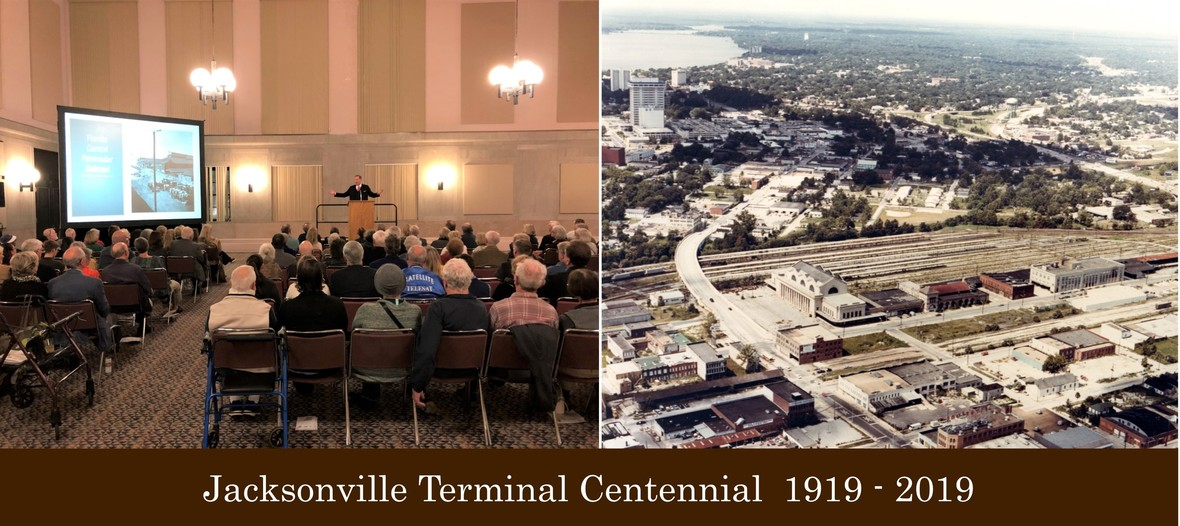/* styles */ The Historical Society celebrated the centennial of the Jacksonville Terminal, Monday, November 18. Alan Bliss, JHS Executive Director, and Guest Speaker, Clarence Gooden, shared the history and impact of the terminal in Jacksonville's cultural and economic development. After serving as a thriving Southeastern transportation hub for over fifty years, the terminal lapsed into disrepair and neglect. It was rescued by business leaders and the City of Jacksonville, and re-opened as the Prime F. Osborn III Convention Center in 1986. Thank you to Prime Osborn Facility Manager, Jim Pritchard, ASM Global staff and PRI Productions for their ongoing care of this great facility and their assistance with the centennial celebration.
 table div table+table+table+table+table+table+table+table+table+table+table+table+table+table+table+table+table+table+table+table+table+table+table+table+table+table+table+table+table+table+table+table+table+table+table+table+table+table+table+table+table+table+table+table div table{width:100%;padding:0}table div table+table+table+table+table+table+table+table+table+table+table+table+table+table+table+table+table+table+table+table+table+table+table+table+table+table+table+table+table+table+table+table+table+table+table+table+table+table+table+table+table+table+table+table div table img{width:96.23%;padding:0;float:none}table div table+table+table+table+table+table+table+table+table+table+table+table+table+table+table+table+table+table+table+table+table+table+table+table+table+table+table+table+table+table+table+table+table+table+table+table+table+table+table+table+table+table+table+table div table td{width:100%;padding:0 1.88% 18px}/* styles */## Coming in 2020!The 2020 Speaker Series and more

 /* styles */ Plans for the 2020 Speaker Series are underway. Stay tuned to the newsletter and website as we announce dates for these popular events. The Jacksonville Historical Society Speaker Series is sponsored by Retina Associates, Fred H. Lambrou, Jr., M.D.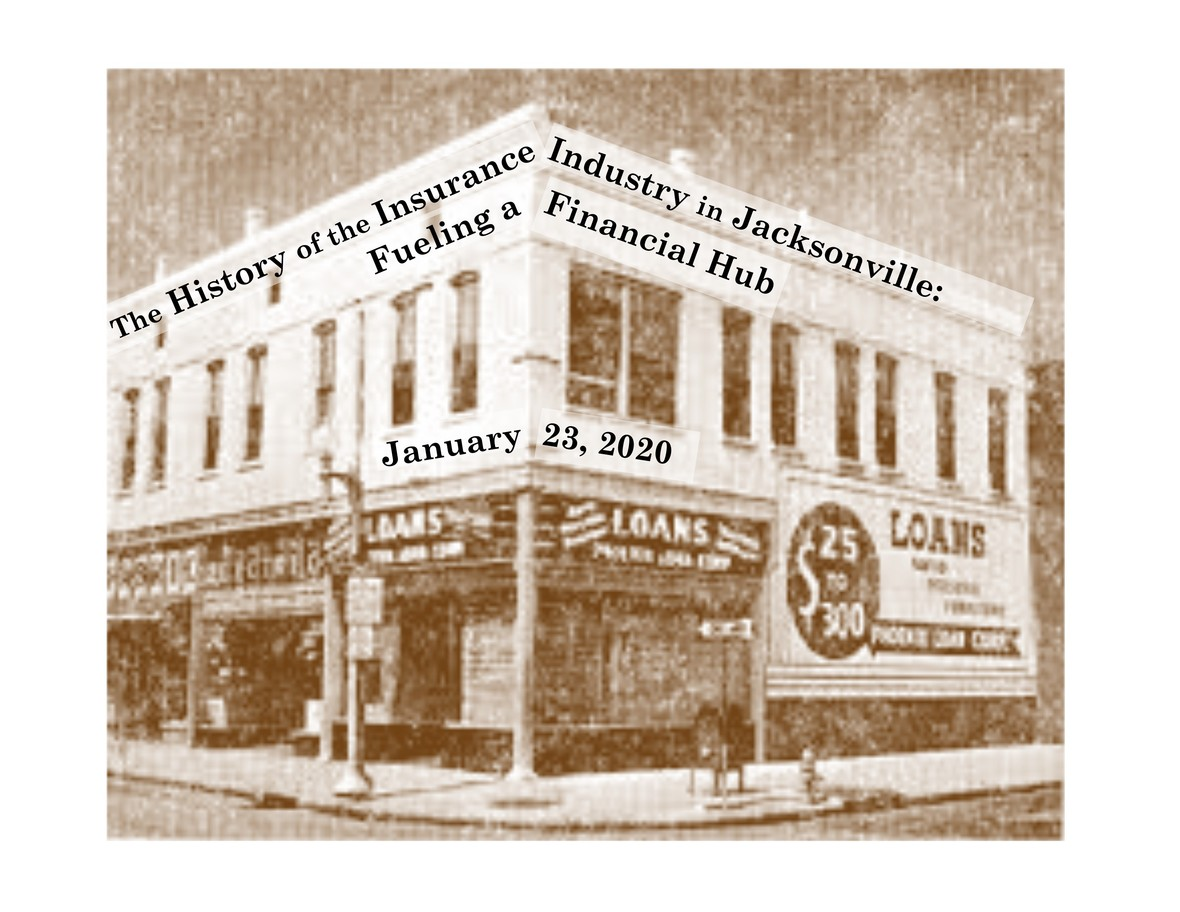# JANUARY 2020: Fueling a Financial Hub: The Insurance Industry in Jacksonville

Insurance industry executive and JHS board member, J. F. Bryan IV, will recount Jacksonville's role in the mid-twentieth century insurance boom.
When: Thursday, January 23, 2020 6:30pm
Where: Old St. Andrews - 317 A. Philip Randolph Blvd.
JHS Members: Free
Guests and Non-members: Suggested donation \$10

 table div table+table+table+table+table+table+table+table+table+table+table+table+table+table+table+table+table+table+table+table+table+table+table+table+table+table+table+table+table+table+table+table+table+table+table+table+table+table+table+table+table+table+table+table+table+table+table+table+table div table{width:100%;padding:0}table div table+table+table+table+table+table+table+table+table+table+table+table+table+table+table+table+table+table+table+table+table+table+table+table+table+table+table+table+table+table+table+table+table+table+table+table+table+table+table+table+table+table+table+table+table+table+table+table+table div table img{width:96.23%;padding:0;float:none}table div table+table+table+table+table+table+table+table+table+table+table+table+table+table+table+table+table+table+table+table+table+table+table+table+table+table+table+table+table+table+table+table+table+table+table+table+table+table+table+table+table+table+table+table+table+table+table+table+table div table td{width:100%;padding:0 1.88% 18px}/* styles */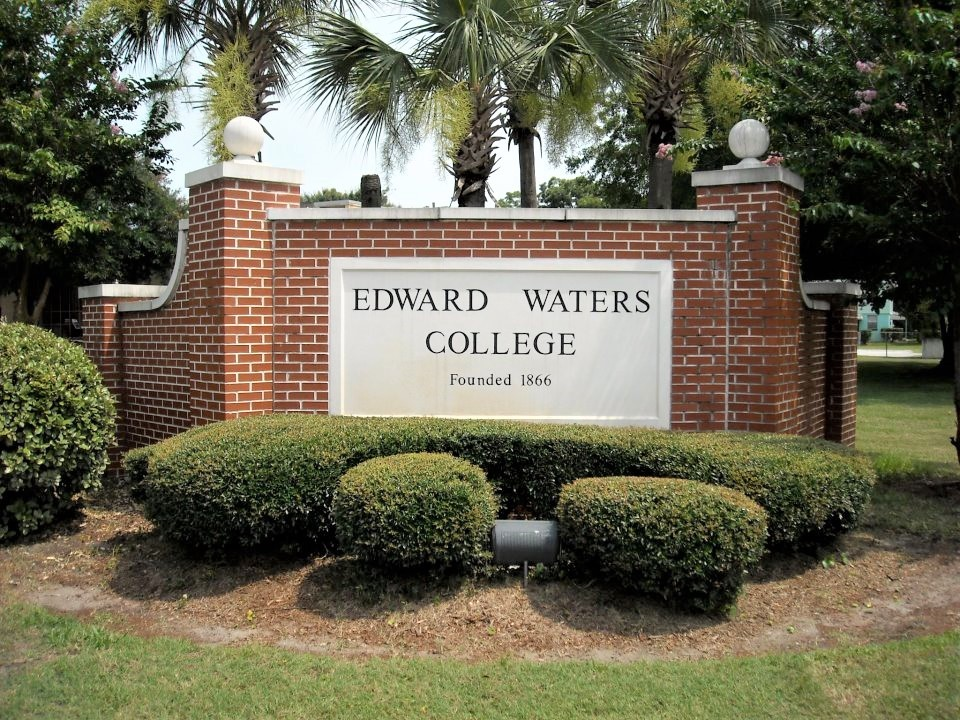# FEBRUARY 2020: The First College: 154 Years of Jacksonville’s Edward Waters College

Dr. David Jamison, Professor of History, will explore the history of Edward Waters College in its 154th year of operation as Florida’s oldest independent institution of higher learning as well as the state’s first institution established for the education of African Americans.
When: February - Date to be announced
Where: Edward Waters College - 1658 Kings Road Jacksonville, FL 32209

 table div table+table+table+table+table+table+table+table+table+table+table+table+table+table+table+table+table+table+table+table+table+table+table+table+table+table+table+table+table+table+table+table+table+table+table+table+table+table+table+table+table+table+table+table+table+table+table+table+table+table+table+table div table{width:100%;padding:0}table div table+table+table+table+table+table+table+table+table+table+table+table+table+table+table+table+table+table+table+table+table+table+table+table+table+table+table+table+table+table+table+table+table+table+table+table+table+table+table+table+table+table+table+table+table+table+table+table+table+table+table+table div table img{width:96.23%;padding:0;float:none}table div table+table+table+table+table+table+table+table+table+table+table+table+table+table+table+table+table+table+table+table+table+table+table+table+table+table+table+table+table+table+table+table+table+table+table+table+table+table+table+table+table+table+table+table+table+table+table+table+table+table+table+table div table td{width:100%;padding:0 1.88% 18px}/* styles */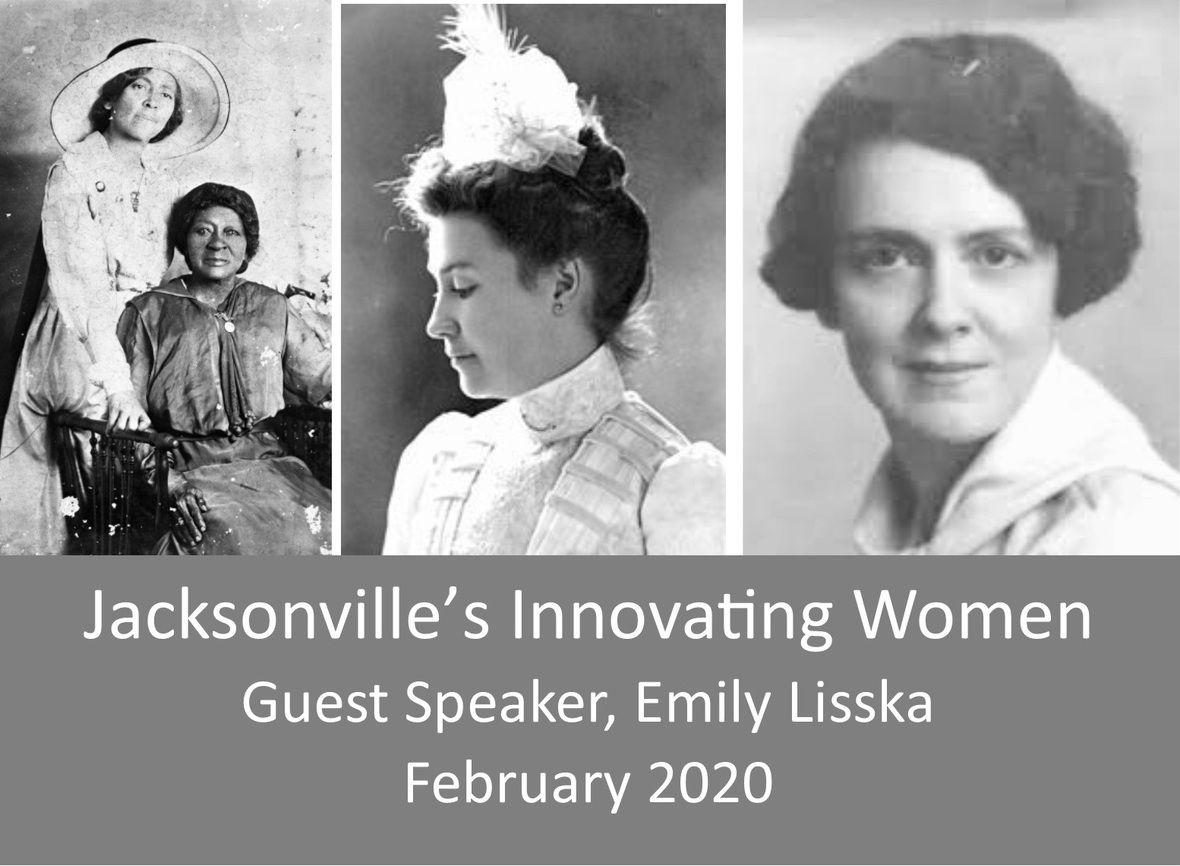# MARCH 2020: Jacksonville's Innovating Women

Emily Lisska, former director of the Jacksonville Historical Society, will share the accomplishments of the some of the remarkable women who have impacted our community. Ms. Lisska continues to lead in historic preservation initiatives as president of the Florida Historical Society, board member of the Metropolitan Park Association and instructor at UNF's Osher Life Learning Institute.
When: March 2020 - Date to be announced
Where: Old St. Andrews - 317 A. Philip Randolph Blvd.

 table div table+table+table+table+table+table+table+table+table+table+table+table+table+table+table+table+table+table+table+table+table+table+table+table+table+table+table+table+table+table+table+table+table+table+table+table+table+table+table+table+table+table+table+table+table+table+table+table+table+table+table+table+table+table+table div table{width:100%;padding:0}table div table+table+table+table+table+table+table+table+table+table+table+table+table+table+table+table+table+table+table+table+table+table+table+table+table+table+table+table+table+table+table+table+table+table+table+table+table+table+table+table+table+table+table+table+table+table+table+table+table+table+table+table+table+table+table div table img{width:96.23%;padding:0;float:none}table div table+table+table+table+table+table+table+table+table+table+table+table+table+table+table+table+table+table+table+table+table+table+table+table+table+table+table+table+table+table+table+table+table+table+table+table+table+table+table+table+table+table+table+table+table+table+table+table+table+table+table+table+table+table+table div table td{width:100%;padding:0 1.88% 18px}/* styles */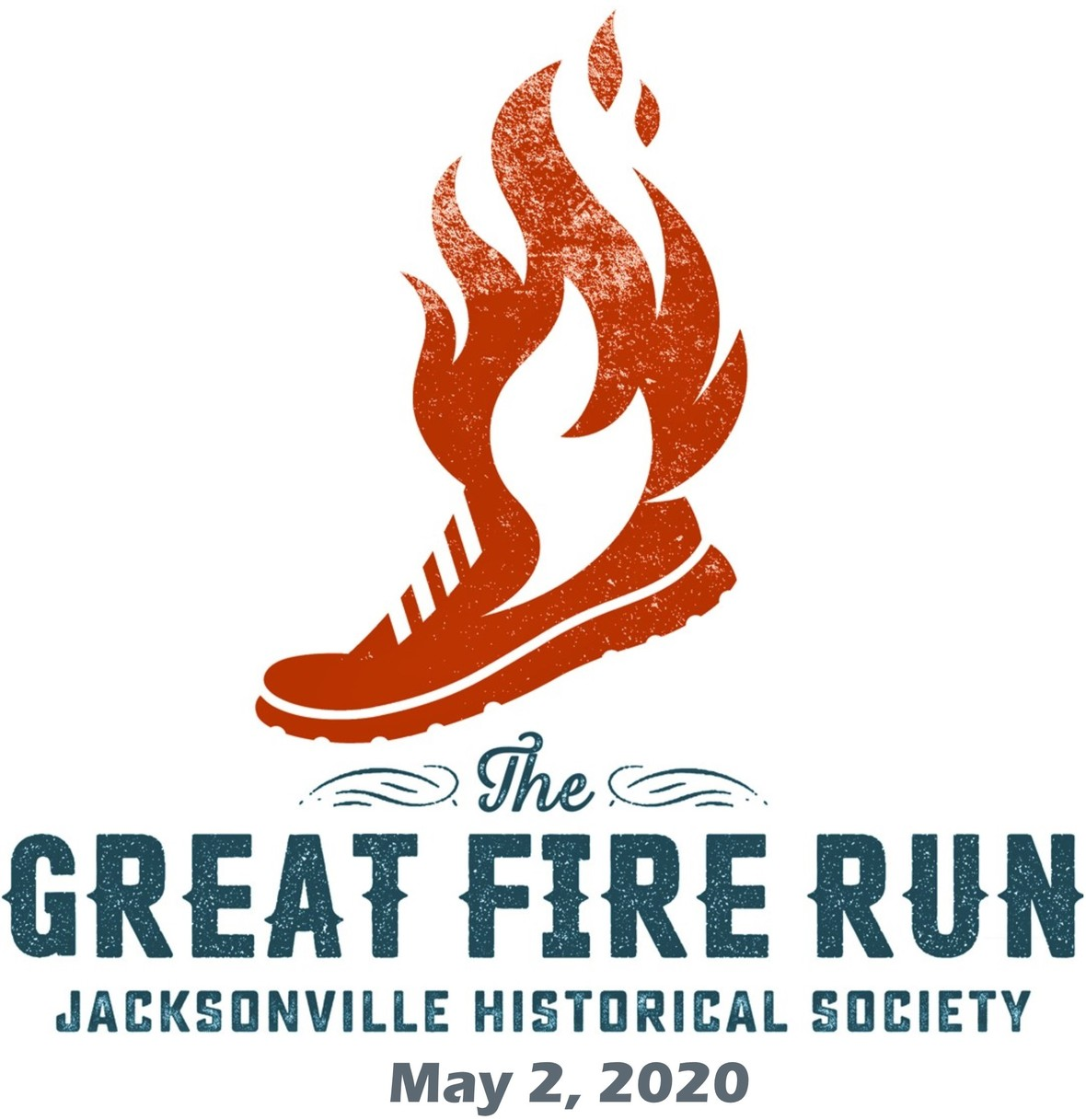# The Great Fire Run - May 2, 2020

The Jacksonville Fire Department and fans will cheer you on as you run the outline of the May 3, 1901 that destroyed 146 city blocks, 1,700 homes and 2,368 buildings in downtown Jacksonville. Enjoy the morning downtown with post-race activities for the whole family.
Early registration available until December 31st.

 table div table+table+table+table+table+table+table+table+table+table+table+table+table+table+table+table+table+table+table+table+table+table+table+table+table+table+table+table+table+table+table+table+table+table+table+table+table+table+table+table+table+table+table+table+table+table+table+table+table+table+table+table+table+table+table+table+table+table+table div table{width:100%;padding:0}table div table+table+table+table+table+table+table+table+table+table+table+table+table+table+table+table+table+table+table+table+table+table+table+table+table+table+table+table+table+table+table+table+table+table+table+table+table+table+table+table+table+table+table+table+table+table+table+table+table+table+table+table+table+table+table+table+table+table+table div table img{width:96.23%;padding:0;float:none}table div table+table+table+table+table+table+table+table+table+table+table+table+table+table+table+table+table+table+table+table+table+table+table+table+table+table+table+table+table+table+table+table+table+table+table+table+table+table+table+table+table+table+table+table+table+table+table+table+table+table+table+table+table+table+table+table+table+table+table div table td{width:100%;padding:0 1.88% 18px}/* styles *//* styles */ The Jacksonville Historical Society has an opening for a skilled Office Administrator: Office Administrator Job Responsibilities If you would like to join this important organization, send your resume and cover letter to Alan Bliss, Executive Director, alan.bliss@jaxhistory.org.
 table div table+table+table+table+table+table+table+table+table+table+table+table+table+table+table+table+table+table+table+table+table+table+table+table+table+table+table+table+table+table+table+table+table+table+table+table+table+table+table+table+table+table+table+table+table+table+table+table+table+table+table+table+table+table+table+table+table+table+table+table+table+table div table{width:100%;padding:0}table div table+table+table+table+table+table+table+table+table+table+table+table+table+table+table+table+table+table+table+table+table+table+table+table+table+table+table+table+table+table+table+table+table+table+table+table+table+table+table+table+table+table+table+table+table+table+table+table+table+table+table+table+table+table+table+table+table+table+table+table+table+table div table img{width:96.23%;padding:0;float:none}table div table+table+table+table+table+table+table+table+table+table+table+table+table+table+table+table+table+table+table+table+table+table+table+table+table+table+table+table+table+table+table+table+table+table+table+table+table+table+table+table+table+table+table+table+table+table+table+table+table+table+table+table+table+table+table+table+table+table+table+table+table+table div table td{width:100%;padding:0 1.88% 18px}/* styles */## Our Mission:

To educate and inspire the greater Jacksonville community to value its history, by fostering understanding of how the region's past shapes our present.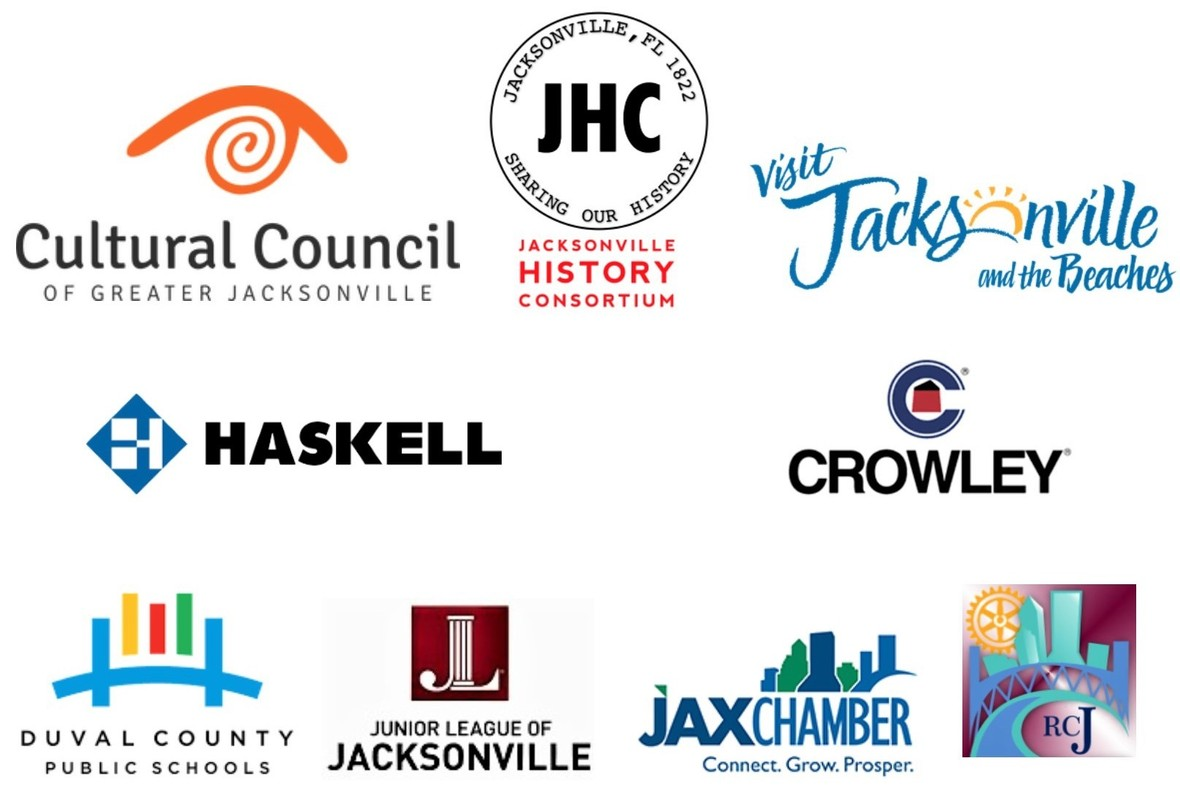## JHS is thankful for the many organization that support us in our mission!

 table div table+table+table+table+table+table+table+table+table+table+table+table+table+table+table+table+table+table+table+table+table+table+table+table+table+table+table+table+table+table+table+table+table+table+table+table+table+table+table+table+table+table+table+table+table+table+table+table+table+table+table+table+table+table+table+table+table+table+table+table+table+table+table+table+table+table div table{width:100%;padding:0}table div table+table+table+table+table+table+table+table+table+table+table+table+table+table+table+table+table+table+table+table+table+table+table+table+table+table+table+table+table+table+table+table+table+table+table+table+table+table+table+table+table+table+table+table+table+table+table+table+table+table+table+table+table+table+table+table+table+table+table+table+table+table+table+table+table+table div table img{width:96.23%;padding:0;float:none}table div table+table+table+table+table+table+table+table+table+table+table+table+table+table+table+table+table+table+table+table+table+table+table+table+table+table+table+table+table+table+table+table+table+table+table+table+table+table+table+table+table+table+table+table+table+table+table+table+table+table+table+table+table+table+table+table+table+table+table+table+table+table+table+table+table+table div table td{width:100%;padding:0 1.88% 18px}/* styles */## Staff:

Alan Bliss, Ph.D. , Executive Director | Mitch Hemann, Archivist | Susan Prattos, Administrator | Imani Phillips, Archives & Office Assistant | Sherrard Ceglia, Archives Assistant | David Woodard, Facilities Manager